# Motion in a Plane Class 11 NCERT solutions

Question 4.1
State, for each of the following physical quantities, if it is a scalar or a vector: volume, mass, speed, acceleration, density, number of moles, velocity, angular frequency, displacement, angular velocity.
Solution.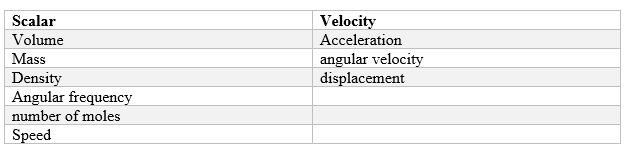Question 4.2
Pick out the two scalar quantities in the following lists: force, angular momentum, work, current, linear momentum, electric field, average velocity, magnetic moment, relative velocity.
Solution.
The only scalar quantities in the given list are Work and Current

Question 4.3
Pick out the only vector quantity in the following lists: temperature, pressure, impulse, time, power, total path- length, energy, gravitational potential, coefficient of friction, charge.
Solution.
Here impulse is the only vector quality in the list.

Question 4.4
State with reasons, whether the following algebraic operations with scalar and vector physical quantities are meaningful.
(b) adding a scalar to a vector of the same dimensions
(c) multiplying any vector by any scalar
(d) multiplying ant two scalars
(f) adding a component of a vector to the same vector.
Solution.
(a) The algebraic option would not be meaningful as only the scalars of same dimensions can be added.
(b) The algebraic option would not be meaningful as a scalar cannot be added to a vector.
(c) This is meaningful as multiplying a vector with a scalar gives the scalar (number) times the vector quantity .We gets a bigger vector. For example, when acceleration $\vec{A}$ is multiplied by mass $m$, we get a force $\vec{F} = m \vec{A}$.
(d) This is correct as two scalars multiplied yield a meaningful result, for example multiplication of speed and time provides the distance travelled by the object.
(e) The algebraic option would not be meaningful as the two vectors of same dimensions can be added.
(f) This is also meaningless.

Question 4.5
Read each statement below carefully and state with reasons, if it is true or false:
(a) The magnitude of a vector is always a scalar
(b) Each component of a vector is always a scalar.
(c) The total path length is always equal to the magnitude of the displacement vector of a particle.
(d) The average speed of a particle (defined as total path length divided by the time taken to cover the path) is either greater or equal to the magnitude of average velocity of the particle over the same interval of time.
(e) Three vectors not lying in plane can never add up to give a null vector.
Solution.
(a) True
(b) False as each component is itself a vector.
(c) False. This is true only the particle moves along a straight line in the same direction.
(d) True, as the total path length is either greater than or equal to the magnitude of the displacement vector.
(e) True, as they cannot represent the three sides of a triangle taken in the same order.

Question 4.6
Establish the following vector inequalities geometrically or otherwise: \begin{align*} \left( a \right) \left| \vec{A}+\vec{B} \right|\le \left| \vec{A} \right|+\left| \vec{B} \right|\\ \left( b \right) \left| \vec{A}+\vec{B} \right|\ge \left| \vec{A} \right|-\left| \vec{B} \right|\\ \left( c \right) \left| \vec{A}-\vec{B} \right|\le \left| \vec{A} \right|+\left| \vec{B} \right|\\ \left( d \right) \left| \vec{A}-\vec{B} \right|\le \left| \vec{A} \right|-\left| \vec{B} \right| \end{align*} When does the equality sign above apply?
Solution.
We draw the vectors $\vec{A}$ and $\vec{B}$ and find the addition and subtraction using the parallelogram method as shown in Below figure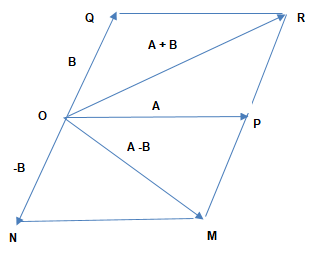$OP =\left| \vec{A} \right|$
$OQ = \left| \vec{B} \right|$
$ON = \left| \vec{-B} \right|$
and
$OR = \left| \vec{A}+\vec{B} \right|$
$OM = \left| \vec{A}-\vec{B} \right|$
(a) to prove $\left| \vec{A}+\vec{B} \right|\le \left| \vec{A} \right|+\left| \vec{B} \right|$
Now We know that the length of one side of triangle is always less than the sum of the lengths of the other two sides. Hence from, $\Delta OPR$ we have
$OR < OP+ PR$
or,
$OR < OP+ OQ$
or,
$\left| \vec{A}+\vec{B} \right|\le \left| \vec{A} \right|+\left| \vec{B} \right| \qquad \left( 1 \right)$
Now if the both the vectors are acting in same direction ,then
$\left| \vec{A}+\vec{B} \right| = \left| \vec{A} \right|+\left| \vec{B} \right| \qquad \left( 2 \right)$
From 1 and 2
$\left| \vec{A}+\vec{B} \right|\le \left| \vec{A} \right|+\left| \vec{B} \right|$

(b)
$\left| \vec{A}+\vec{B} \right|\ge \left| \vec{A} \right|-\left| \vec{B} \right|$
From $\Delta OPR$ , We have,
$OR + PR > OP$
or, $OR > \mid OP - PR \mid$
Now Since $PR = OQ$
$OR > \mid OP - OQ \mid$
Hence
$|\vec A + \vec B| > ||\vec A| - |\vec B|| \qquad (3)$
If the two vectors $\vec A$ and $\vec B$ are acting along a straight line in opposite directions, then
$|\vec A + \vec B| = ||\vec A| - |\vec B|| \qquad (4)$
From 3 and 4
$|\vec A + \vec B| \ge ||\vec A| - |\vec B||$

(c) $|\vec{A}-\vec{B}| \le | \vec{A}| + | \vec{B}|$
Now We know that the length of one side of triangle is always less than the sum of the lengths of the other two sides
In $\Delta OPM$ we have
$OM < OP + PM$
$OM < OP + ON$
or
$|\vec{A}-\vec{B}| < | \, | \vec{A} | + | \vec{-B} |\,|$
or
$| \vec{A} -\vec{B} | < |\,| \vec{A} | + | \vec{B}|\,| \qquad \left( 5 \right)$
If the two vectors are acting along the straight line but in opposite direction then,
$| \vec{A} -\vec{B} | = |\,| \vec{A} | + | \vec{B} |\,| \qquad \left( 6 \right)$
From 5 and 6
$|\vec{A} -\vec{B}| \le | \vec{A}| + | \vec{B} |$

(d) $| \vec{A}-\vec{B}| \ge |\,| \vec{A} | - | \vec{B}|\,|$
In Figure, from $\Delta OPM$ we have,
$OM+PM > OP$ or, $OM > | OP - PM|$
or,
$OD > | OP - ON |$
$| \vec{A}-\vec{B} | \ge | \vec{A} | - | \vec{B} | \qquad \left( 7 \right)$
If two vectors $\vec{A}$ and $\vec{B}$ are along the same straight line in the same direction then,
$| \vec{A}-\vec{B} | =| \vec{A} | - | \vec{B} | \qquad \left( 8 \right)$
From 7 and 8
$| \vec{A}-\vec{B} | \ge | \, | \vec{A} | - | \vec{B} |\, |$

Question 4.7
Given $\vec{A}+\vec{B}+\vec{C}+\vec{D}=0,$ which of the following statements are correct?
(a) $A, B, C \; \text{and} \;D$ must be a null vector
(b) The magnitude of $\vec A +\vec C$ equals the magnitude of $\vec{B}+\vec{D}.$
(c) The magnitude of $\vec A$ can never be greater than the sum of the magnitudes of $\vec B, \vec C$ and $\vec D$
(d) $\vec B +\vec C$ must lie in the plane of$\vec A+\vec D$, if $\vec A$ and $\vec D$ are not collinear and in the line of $\vec A$ and $\vec D$, if they are collinear.
Solution.
(a) Incorrect, because $\vec{A}+\vec{B}+\vec{C}+\vec{D}$ can be zero in many ways other than $\vec{A},\vec{B}, \vec{C}$ and $\vec{D}$ must each be null vector.
(b) This is correct
Reason $\vec{A}+\vec{B}+\vec{C}+\vec{D}=0$ therefore, $\vec{A}+\vec{C}=-(\vec{B}+\vec{D})$ Taking modulus on each side $|\vec{A}+\vec{C}|= |\vec{B}+\vec{D}|$
Hence the magnitude of $\vec A +\vec C$ equals the magnitude of $\vec{B}+\vec{D}.$
(c) This is correct
Reason
$\vec{A}+\vec{B}+\vec{C}+\vec{D}=0$
or
$\vec{A}=-(\vec{B}+\vec{C}+\vec{D})$
It means the magnitude of $\vec A$ is equal to the magnitude of vector $\vec B+\vec C+\vec D$. Since the sum of the magnitude of $\vec B, \vec C \text{ and } \vec D$ may be equal or greater than the magnitude of hence the magnitude of $vec A$ can never be greater than the sum of the magnitude of $\vec B, \vec C \text{ and } \vec D$ .
(d) This is correct
Reason
$\vec{A} +\vec{B}+\vec{C}+\vec{D}=0$
or $\vec{A}+\left( \vec{B}+\vec{C} \right) +\vec{D}=0$.

The resultant sum of the three vectors $\vec{A}$, $\vec{B}+\vec{C})$, $\vec{D}$ can be zero only} if $(\vec{B}+\vec{C})$ lies in the plane of $\vec{A}$ and $\vec{D}$and these three vectors are represented by the three sides of the triangle taken in one order. If $\vec{A}$ and $\vec{D}$ are collinear, then $(\vec{B}+\vec{D})$ must be in the line of $\vec{A}$ and $\vec{D}$, only then vector sum of all the vectors will be zero.

Question 4.8
Three girls skating on a circular ice ground of radius 200 m start from a point P on the edge of the ground and reach a point Q diametrically opposite to P following different paths as shown in Fig. What is the magnitude of the displacement vector for each? For which girl is this equal to the actual length of path skate?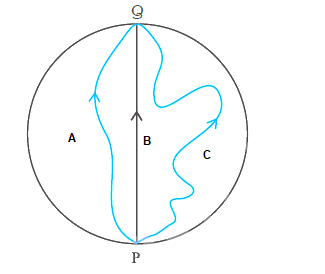Solution.
Displacement for each girl =Vector Joining start point and End point= $\vec {PQ}$ .
Therefore, magnitude of the displacement for each girl = $\vec{PQ}$ = diameter of circular ice ground$= 2\times 200=400 m$.
It is very apparent from the figure
For Girl B, the magnitude of displacement = length of path skate.

Question 4.9
A cyclist starts from the centre O of a circular park of radius 1 km, reaches the edge P of the park, then cycles along the circumference, and returns to the centre along QO as shown in Fig. If the round trip takes 10 min, what is the
(a) net displacement,
(b) average velocity, and
(c) average speed of the cyclist.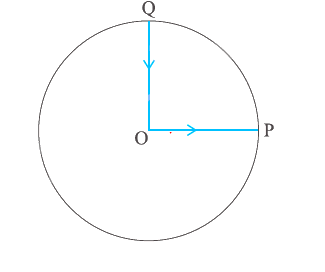Solution.
(a) Since both the start and final positions are the same therefore the net displacement is zero.
(b) Average velocity is define as the ratio of net displacement and total time taken. Since the net displacement is zero therefore the average velocity is also zero. (c) Average speed is defined as the ratio of total distance travelled and total time taken
\begin{align*} \text{Average speed }&=\frac{\text{distance covered}}{\text{time taken}}\\ &=\frac{OP+\text{Actual distance }PQ+QO}{\text{10 }minute}\\ &=\frac{\text{1km }+\left( \frac{1}{4}\times 2\pi \times \text{1km } \right) +\text{1km}}{\frac{10}{60h}}\\ &=6\left( 2+\frac{22}{14} \right) \text{km h}^{-1}=6\times \frac{50}{14}\text{km h}^{-1}\\ &=\text{21.43 km h}^{-1} \end{align*}
Question 4.10
On an open ground, a motorist follows a track that turns to his left by an angle of 60° after every 500 m. Starting from a given turn, specify the displacement of the motorist at the third, sixth and eighth turn. Compare the magnitude of the displacement with the total path length covered by the motorist in each case.
Solution.
In this question, the path is a regular hexagon $PQRSTU$ of side length $500\, m$. In Fig,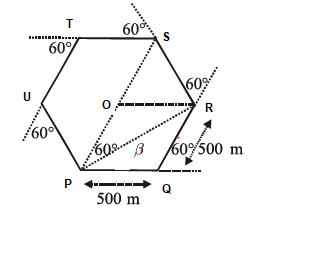Let the motorist start from point $P$.
His points after each turn would be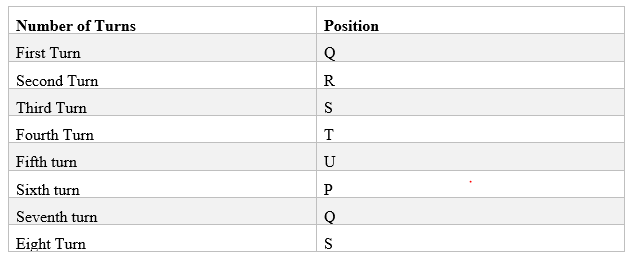Now ,lets find the displacement and total path lenght(distance) in the asked turns Third Turn
The motorcyclist will take the $3^{rd}$ turn at $S$.
Displacement vector at $S = PS$
Magnitude of this displacement $=PO + OS=500+500 = 1000m$
Total path length from $P$ to $S$ $= PQ + QR + RS = 500+500+500 = 1500m$
Sixth Turn
The motorcyclist will take the $6^{th}$ turn at $P$.
Therefore, Displacement vector is null vector.
Total path length(distance) $= PQ + QR + RS+ ST + TU$
or, Total Path $= 500+500+500+500+500+500=3000m$
Eighth Turn
The motorcyclist takes the $8^{th}$ turn at $R$.
Therefore, Displacement vector $= PR$, with is represented by the diagonal of the parallelogram $PQRO$. Now this displacement can be found using the law of cosines which states as below $PR= \sqrt {PQ^2 + QR^2 + 2 PQ QR cos \theta}$
$\beta = tan^{-1} \frac {QR sin \theta}{PQ + QR cos \theta}$
$PR=\sqrt{\left[ \left( 500 \right) ^2+\left( 500 \right) ^2+2\times \left( 500 \right) \times \left( 500 \right) \cos 60^0 \right]}$
$=\left[ \left( 500 \right) ^2+\left( 500 \right) ^2+250000 \right] =\text{866.03 }m$
$\tan \beta =\text{500 }\sin 60^0/ \left[ \text{500 }+\text{500 }\cos 60^0 \right]$
$=\left( 500\sqrt{3}/ 2 \right) / \left[ \text{500 }\left( \text{1 }+\text{1/}2 \right) \right] =\text{1/}\sqrt{3}$
$=\tan 30^0$
$\beta =30^0$
Now total path lenght = 3000 + 500 + 500 =4000 m
In Short, here are the answer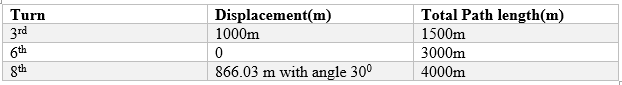Question 4.11
A passenger arriving in a new town wishes to go from the station to a hotel located 10 km away on a straight road from the station. A dishonest cabman takes him along a circuitous path 23 km long and reaches the hotel in 28 minutes. What is
(a) the average speed of the taxi,
(b) the magnitude of average velocity? Are the two equal?
Solution.
Here,actual path length travelled, $s = 23 km,$ Displacement = 10 km,
Time taken,$t = 28 \min = \frac{28}{60}h.$
(a) $\text{Average speed of the taxi} = \frac{{actual path length}}{\text{time taken}} = \frac{23}{28/60}= 49.3 km/h$ (b) $\text{Magnitude of average velocity} = \frac{\text{displacement}}{\text{time taken}} =\frac{10}{28/60} = 21.4 km/h$ The,average speed is not equal to the magnitude of average velocity.

Question 4.12
Rain is falling vertically with a speed of$30m/s$. A woman rides a bicycle with a speed of $10 m/s$ in the north to south direction. What is the direction in which she should hold her Umbrella?
Solution.
The situation is described in below figure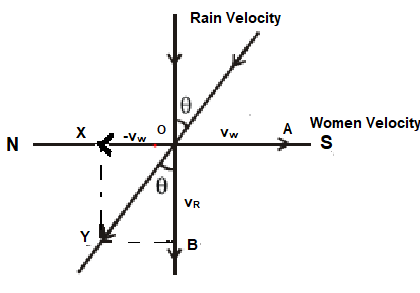The rain is falling along OA with speed $30\,m/s$
Woman rider is moving along OB with $10\,m/s$
i.e. $OA=30\,m/s$ and $OB=10\,m/s$.
The woman rider can protect herself from the rain if she holds her umbrella in the direction of relative velocity of rain w.r.t. woman.
Now relative velocity of rain w.r.t. woman is given by vector $\mathbf{v_R} - \mathbf{v_w}$.
Now this relative velocity vector can be represented by the line OY in the parallelogram OXYB using parallologram method of vector addition and subtraction
If $\angle BOY = \theta ,$

then in $\Delta OYB,$
\begin{align*} \tan \theta & = \frac{YB}{OB}\\ & =\frac{OX}{OY} \\ & = \frac {10}{30} \\ & = 0.3333 = \tan 18^{\circ} 26' \end{align*} So she should hold her Umbrella at an angle $\beta = 18^{\circ} 26'$ with vertical in forward direction.

Question 4.13
A man can swim with a speed of 4.0 km/h in still water. How long does he take to cross a river 1.0 km wide if the river flows steadily at 3.0 km/h and he makes his strokes normal to the river current? How far down the river does he go when he reaches the other bank?
Solution.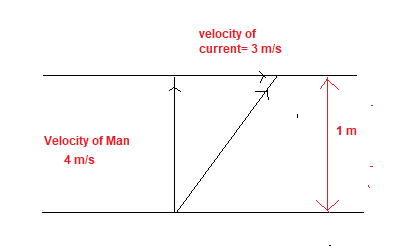Time to cross the river,
$t = \frac{\text{width of river}}{\text{speed of man}}$
$t = \frac{1 km}{4 km/h} = \frac{1}{4} h = 15 m.$
Distance moved along the river in time $t$ would be
$t = v_r\times t = 3 km/h \times \frac{1}{4} h = 750 m$

Question 4.14
In a harbour, wind is blowing at the speed of 72 km/h and the flag on the mast of a boat anchored in the harbour flutters along the N-E direction. If the boat starts moving at a speed of 51 km/h to the north, what is the direction of the flag on the mast of the boat.
Solution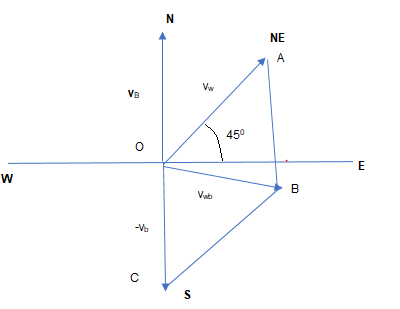When the boat is at rest in the harbour, the flag flutters along the N-E direction which means velocity of wind is along the north-east direction.

Wind velocity is represented by vector $\vec{v}_W$ in the above figure
$|\vec{v}_W|=72$ km/h

Now when the boat is moving, the flag will flutters in the direction of wind with respect to Boat
Boat velocity is represented by the vector $\vec{v}_B$ in the above figure
$|\vec{v}_B|=51$ km/h

Now the relative velocity of wind with respect to Boat is given by
$\vec{v}_{WB} = \vec{v}_W - \left( \vec{v}_B \right)$
or
$\vec{v}_{WB} = \vec{v}_W + \left( - \vec{v}_B \right)$
Now this vector can by the line OB in the parallelogram OABC using parallologram method of vector addition and subtraction
Angle between $\vec{v}_W$ and $- \vec{v}_B$ is $135^0$ i.e.,
$\theta = 135^0$.

Now
Now angle $\angle AOB =\beta$ can be found using the law of cosines which states as below
$tan \beta = \frac {OC sin \theta}{OA + OC cos \theta}$

$\tan \beta =\frac{51 sin 135^0}{72+51 \cos 135^0}=\frac{51 \sin45^0}{72+51\left( -\cos 45^0 \right)}$
$=\frac{51 \times \left( \frac{1}{\sqrt{2}} \right)}{72 - 51 \left( \frac{1}{\sqrt{2}} \right)} = 1.0039$
$\therefore b = \tan ^{-1} \left( 1.0039 \right) = 45.1^0$
Angle w.r.t. east direction $= 45.1^0 - 45^0 = 0.1^0$
It means the flag will flutter almost due east.

Question 4.15
The ceiling of a long hall is 25m high. What is the maximum horizontal distance that a ball thrown with a speed of $40 m/s$ can go without hitting the ceiling of the hall?
Solution
Given, $\mu =40ms^{-1},H=25 m$
Let,$\theta =$ the angle of projection with the horizontal direction
We know that Height reached and Range is given by
$H=\frac {u^2 sin^2 \theta}{2g}$
$R= \frac {u^2 sin2 \theta}{g}$

We can see that Height and Range both depends on initial velocity and angle of projection. Height increase with the angle of projection.But we have been given,here that maximum height reached $=25\,m$

Therefore, $H=25=\frac{\mu ^2\sin ^2\theta}{2g}=\frac{\left( 40 \right) ^2\sin ^2\theta}{\left(2 \times 9.8 \right)}$
$\sin \theta =\left( \frac{25\times 2\times 9.8}{40^2} \right) ^{1/2}=0.55334 =\sin 333.6^0$
or,$\theta =33.6^0$
Now Horizontal range or maximum Horizontal distance,
$R=\frac{\mu ^2\sin ^2\theta}{g}= \frac{\left( 40 \right) ^2\sin \left(2 \times 33.6^0 \right)}{9.8} = 150.5 m$

Question 4.16
A cricketer can throw a ball to a maximum horizontal distance of 100m. How much high above the ground can the cricketer throw the same ball?
Solution

Let $\mu =$velocity of projection of the ball.
We know that Height reached and Range is given by
$H=\frac {u^2 sin^2 \theta}{2g}$
$R= \frac {u^2 sin2 \theta}{g}$
The ball will cover maximum horizontal distance when angle of projection with horizontal,
$\theta =45^0$.
Then $R_{\max}=\frac{\mu ^2}{g}$
So,
$100 = \frac {u^2}{g}$ --(1)
Now maximum height can be reached when the $\theta =90^0$, i,e vertically upward direction
So,
$H= \frac {u^2 sin^2 \theta}{2g}=\frac {u^2}{2g}$
Now from equation (1),we get
H= 50 m
Question 4.17
A stone tied to the end of a string 80cm long is whirled in a horizontal circle with a constant speed. If the stone makes 14 revolutions in 25 s what is the magnitude and direction of acceleration of the stone?
Solution

Given,
$r=80cm=0.8m,n=\frac{14}{25}s^{-1}$,
$\omega =2\pi \nu =2\times \frac{22}{7}\times \frac{14}{25}=\frac{88}{25}rad.s^{-1}$
Centripetal acceleration is given by,
$a = (\omega)^2 r= (\frac {88}{25})^2 \times .8 = 9.91 m/s^2$

Question 4.18
An aircraft executes a horizontal loop of radius 1.00 km with a steady speed of 900 km/h. Compare its centripetal acceleration with the acceleration due to gravity.
Solution
Given,
$r=1km=10^3m$
$v=900kmh^{-1}=900\times \frac{5}{18}=250ms^{-1}$
Centripetal acceleration is given by
$a_c=\frac{v^2}{r}=\frac{\left( 250 \right) ^2}{10^3}=62.5ms^{-2}$
Now,Comparing Centripetal acceleration with acceleration due to gravity
$\frac{a_c}{g}=\frac{62.5}{9.8}=6.38$.

Question 4.19
Read each statement below carefully and state, with reasons, if it is true or false:
(a) The net acceleration of a particle in circular motion is always along the radius of the circle towards the centre.
(b) The velocity vector of a particle at a point is always along the tangent to the path of the particle at that point.
(c) The acceleration vector of a particle in uniform circular motion averaged over one cycle is a null vector.

Solution
(a) False, the net acceleration of a particle in circular motion is along the radius of the circle towards the centre only in uniform circular motion.In non uniform circular motion, object acceleration has two components, one along the radius of the circle and one along tangent at that point.
(b) True, because while leaving the circular path, the particle moves tangentially to the circular path.
(c) True, the direction of acceleration vector in a uniform circular motion is directed towards the centre of circular path. It is constantly changing with time. The averaged over one cycle all these vectors will be a null vector.

Question 4.20
The position of a particle is given by $\vec{r}=3.0t\hat{i}-2.0t^2\hat{j}+4.0\hat{k}\,\,m$ , where $t$ is in seconds and the coefficients have the proper units for $\vec{r}$ to be in meters.
(a) find the $v$ and $\vec{a}$ of the particle?
(b) what is the magnitude and direction of velocity of the particle at $t = 2.0\,\, s$?
Solution
(a) Velocity vector is given by,
\begin{align*}
\vec{v}&=\frac{d\vec{r}}{dt}\\
&=\frac{d}{dt}\left( 3.0t\hat{i}-2.0t^2\hat{j}+4.0\hat{k} \right) \\
&=3.0\hat{i}-4.0t\hat{j}
\end{align*}
Acceleration vector is given by ,
\begin{align*}
\vec{a}&=\frac{dv}{dt}\\
&=\frac{d}{dt}\left( 3.0\hat{i}-4.0t\hat{j} \right)
\end{align*}
(b) At time $t=2s$,
\begin{align*}
\vec{v}=3.0\hat{i}-4.0\times 2\hat{j}=3.0\hat{i}-8.0\hat{j}\\
\end{align*}
Now we know that Magnitude and angle $\theta$ with the x-axis is given by $|v| = \sqrt {v_x^2 + v_y^2}$
$tan \theta =\frac{v_y}{v_x}$
Hence
$|v|=\sqrt{\left[ \left( 3 \right) ^2+\left( -8 \right) ^2 \right]}ms^{-1}\\ =\sqrt{73}=8.54ms^{-1}$
$\tan \theta =\frac{v_y}{v_x}=-\frac{8}{3}=-2.667=-\tan 69.5^0.$
$\therefore \theta =-69.5^0$ Negative sign indicates, the direction of velocity is below x-axis
Question 4.21
A particle starts from the origin at $t=0 s$ with a velocity of $10.0 \hat{ j} m/s$ and moves in the x-y plane with a constant acceleration of $8.0\hat{i}+2.0\hat{j}\,\,m/s^2$
(a) At what time is the x-coordinate of the particle 16 m? What is the y-coordinate of the particle at that time?
(b) What is the speed of the particle at that time?
Solution
Given,$\vec{u}=10.0\hat{j}$ m/s at $t=0$
$\vec{a}=\left( 8.0\hat{i}+2.0\hat{j} \right) ms^{-2}$ Now we know that acceleration is change in velocity per unit time $\vec{a}=\frac{d}{dt}\left( \vec{v} \right) =\left( 8.0\hat{i}+2.0\hat{j} \right) ms^{-2}$
Integrating the above relation with the change of time, from $0$ to $t$, velocity changes from $\vec{u}$ to $\vec{v}$, we get,
$\int \frac{d}{dt}\left( \vec{v} \right) = \int \left( 8.0\hat{i}+2.0\hat{j} \right)$ $\vec{v}-\vec{u}=\left( 8.0\hat{i}+2.0\hat{j} \right) t$
or,
$\vec{v}=\vec{u}+\left( 8.0\hat{i}+2.0\hat{j} \right) t$
Now again velocity is defined as change is position per unit time

$\vec{v}=\frac{d}{dt}\left( \vec{r} \right) or,d\vec{r}=\vec{v}dt$
So,
$d\vec{r}=\left( u+8.0t\hat{i}+2.0t\hat{j} \right) dt$

Integrating the above relation with the change of time, from $0$ to $t$, displacement changes from $0$ to $r$, we get,
$\int d\vec{r}= \int \left( u+8.0t\hat{i}+2.0t\hat{j} \right) dt$
$\vec{r}=\left( \vec{u}t+\frac{1}{2}8.0t\hat{i}+\frac{1}{2}2.0t^2\hat{j} \right)$
or
Now $\vec{r}$ at any point can be written as
$\vec{r}x\hat{i}+y\hat{j}$
So,
$x\hat{i}+y\hat{j}=10t\hat{j}+4t^2\hat{i}+t^2\hat{j}\\ =4t^2\hat{i}+\left( 10t+t^2 \right) \hat{j}$
So x and y coordinates of the object at any point of time are,
$x=4t^2$ and $y=10t+t^2$
Now we have got the velocity and position equation,lets solve the other parts

(a) At
$x=16m$,
$t=\left( \frac{16}{4} \right) ^{\frac{1}{2}}=2s.\\ y=10\times 2+2^2=24m$
(b) Velocity of the particle at time $t$ is
$v=10\hat{j}+8t\hat{i}+2t\hat{j}$,
when, $t=2s$, then,
$v=10\hat{j}+8\times 2t\hat{i}+2\times 2t\hat{j}=16\hat{i}+14\hat{j}\\ Speed=|\vec{v}|=\sqrt {v_x^2 + v_y^2}=\sqrt{16^2+14^2}=21.26ms^{-1}$

Question 4.22
$\hat{i}$ and $\hat{j}$ are unit vectors along x and y axis respectively. What is the magnitude and direction of the vectors $\hat{i}+\hat{j}$ and $\hat{i}-\hat{j}$ ? What are the components of a vector $A=2\hat{i}+3\hat{j}$ along the direction of $\hat{i}+\hat{j}$ and $\hat{i}-\hat{j}$ ?
Solution
(a) Magnitude of $\hat{i}+\hat{j}$
$=|(\hat{i} + \hat{j}| = \sqrt {1^2 + 1^2} = \sqrt {2}$
Let the vector $\hat{i}+\hat{j}$ makes an $\theta$ with $\hat{i}$, then angle can be found using
$tan \theta = \frac {A_y}{A_x} = 1$
or
$\theta =45$
Now similarly,
Magnitude of $\hat{i}-\hat{j}$
$=|(\hat{i} - \hat{j}| = \sqrt {1^2 + 1^2} = \sqrt {2}$
Let the vector $\hat{i}-\hat{j}$ makes an $\alpha$ with $\hat{i}$
$tan \theta = \frac {A_y}{A_x} = -1$
or
$\theta =-45$ as this vector is below x axis.
Now the Unit vector $\hat{a}$ along the direction of $\hat{i}+\hat{j}$ is given by
$\hat{a} = \frac {\hat{i}+\hat{j}}{|\hat{i}+\hat{j}|}= \frac {\hat{i}+\hat{j}}{\sqrt {2}}$
Similarly Unit vector $\hat{b}$ along the direction of $\hat{i}-\hat{j}$ is given by
$\hat{b} = \frac {\hat{i}-\hat{j}}{|\hat{i}-\hat{j}|}= \frac {\hat{i}-\hat{j}}{\sqrt {2}}$

We are given here $A=2\hat{i}+3\hat{j}$
Now $|A|=\sqrt {2^2 + 3^2} = \sqrt {13}$
Angle $\gamma$ withe x-axis
$\gamma =tan ^{-1} \frac {3}{2} =56.31$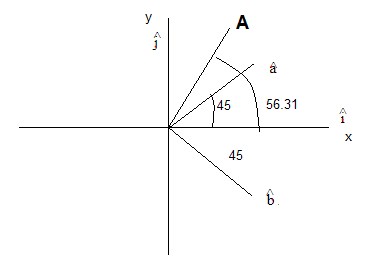We want to express along the two unit vectors $\hat{a}$ and $\hat{b}$
$A=2\hat{i}+3\hat{j} = A_1 \hat{a} + A_2 \hat{b}$
Now $A_1 = |A| cos \alpha$ where $\alpha$ is angle between Vector A and vector $\hat{a}$
Now we can find this using two method
a. Dot Product Method
$A.\hat{a}= |A| cos \alpha$
or

$A_1=|A| cos \alpha= (2\hat{i}+3\hat{j})(\frac {\hat{i}+\hat{j}}{\sqrt {2}}) =\frac {5}{\sqrt {2}}$
b. Calculating the angles graphically. $\alpha =56.31 -45=11.31$
$A_1=|A| cos \alpha=\sqrt {13} cos 11.31 = \sqrt {13} \times .9806$
Similarly $A_2 = |A| cos \beta$ where $\beta$ is angle between Vector A and vector $\hat{b}$ Now we can find this using two method
a. Dot Product Method
$A.\hat{b}= |A| cos \beta$
or
$A_2=|A| cos \beta= (2\hat{i}+3\hat{j})(\frac {\hat{i}-\hat{j}}{\sqrt {2}}) =\frac {-1}{\sqrt {2}}$
b. Calculating the angles graphically. $\beta =56.31 +45=101.31$
$A_2=|A| cos \beta=\sqrt {13} cos 101.31=\frac {-1}{\sqrt {2}}$
So the components are $\frac {5}{\sqrt {2}}$ and $\frac {-1}{\sqrt {2}}$

Question 4.23
For any arbitrary motion in space, which of the following relations are true:
(a) $v_{average}=\left( \frac{1}{2} \right) \left[ v\left( t_1 \right) +v\left( t_2 \right) \right)$
(b) $v_{average}=\frac{\text{[}\left( t_2 \right) -r\left( t_1 \right) \text{]}}{r}\left( t_2-t_1 \right)$
(c) $v\left( t \right) =v\left( 0 \right) +at$
(d) $r\left( t \right) =r\left( 0 \right) +v\left( 0 \right) t+\left( \frac{1}{2} \right) at^2$
(e) $a_{average}=\frac{\left[ v\left( t_2 \right) -v\left( t_1 \right) \right]}{\left( t_2-t_1 \right)}$
( The 'average' stands for a average of the quantity over the time interval $t_1$ to $t_2$ )
Solution
(b) and (e) are true; others are false because relations (a) , (c) and (d) hold only for uniform acceleration.

Question 4.24
Read each statement below carefully and state, with reasons and examples, if it is true or false: A scalar quantity is one that
(a) is conserved in a process
(b) can never take negative values
(c) must be dimensionless
(d) does not vary from one point to another in space
(e) has the same value for observers with different orientations of axes.
Solution
(a) False, because kinetic energy is a scalar but does not remain conserved in an inelastic collision.
(b) False, because potential energy in a gravitational field may have negative values.
(c) False, because mass, length, time, speed, work, etc., all have dimensions.
(d) False, because speed, energy, etc., vary from point to point in space.
(e) True, because a scalar quantity will have the same value for observers with different orientations of axes since a scalar has no direction of its own.

Question 4.25
An aircraft is flying at a height of 3400 m above the ground. If the angle subtended at a ground observation point by the aircraft positions 10.0 s apart is 30°, what is the speed of the aircraft?
Solution
In Fig, $O$ is the Observation point at the ground.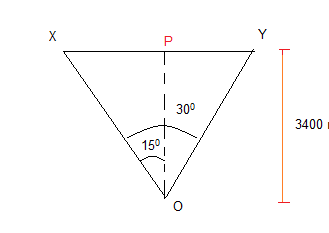$X$ and $Y$ are the positions of aircraft for which $\angle XOY=30^0$.
Draw a perpendicular $OP$ text on $XY$.
Here,$OP=3400m$ and $\angle XOP=\angle POY=15^0$.
In
$\Delta XOP,\,\, XP=OP\tan 15^0\\\ =3400\times 0.2679=910.86m.$
Now $XY=XP+PY=2XP=2\times 910.86m$
Speed of the aircraft,
$v=\frac{distance XY}{time}\\ =\frac{2\times 910.86}{10}=182.17ms^{-1}\\ =182.2ms^{-1}$
Question 4.26
A vector has magnitude and direction. Does it have a location in the space? Can it vary with time? Will two equal vectors a and b at different locations in space necessarily have identical physical effects? Give examples in support of your answer.
Solution
(i) Besides having magnitude and direction, each vector has also a location in space.
(ii) A vector can vary with time. As an example, velocity and acceleration vectors may vary with time.
(iii) Two equal vectors a and b having different locations may not have same physical effect. As an example, two balls thrown with the same force, one from earth and the other from moon will attain different 'maximum heights'.

Question 4.27
A vector has both magnitude and direction. Does it mean that anything that has magnitude and direction is necessarily a vector? The rotation of a body can be specified by the direction of the axis of rotation and the angle of rotation about the axis. Does that make any rotation a vector?
Solution
No. finite rotation of a body about an axis is not a vector because finite rotations do not obey the laws of vector addition.

Question 4.28
Can you associate vectors with (a) the length of a wire bent into a loop (b) a plane area (c) a sphere? Explain.
Solution
(a) We cannot associate a vector with the length of a wire bent into a loop. This is because the length of the loop does not have a definite direction.
(b) We can associate a vector with a plane area. Such a vector is called area vector and its direction is represented by a normal drawn outward to the area.
(c) The area of a sphere does not point in any definite direction. However, we can associate a null vector with the area of the sphere. We cannot associate a vector with
the volume of a sphere.

Question 4.29
A bullet fired at an angle of 30° with the horizontal hits the ground 3.0 km away. By adjusting its angle of projection, can one hope to hit a target 5.0 km away? Assume the muzzle speed to be fixed, and neglect air resistance.
Solution
Here $R=3km=3000m, \theta =30^0, g=9.8ms^{-2}$
As
$R=\frac{u^2\sin 2\theta}{g}\\ \Rightarrow 3000=\frac{u^2\sin 30^0}{9.8}=\frac{u^2\sin 60}{9.8}\\ \Rightarrow u^2=\frac{3000\times 9.8}{\frac{\sqrt{3}}{2}}=3464\times 9.8$
--(1) Also,
$R_m=\frac{u^2\sin 2\theta ^m}{g}\\ \Rightarrow 5000=\frac{3464\times 9.8\times \sin 2\theta ^m}{9.8}$
i.e. $\sin 2\theta ^m=\frac{5000}{3464}=1.44$
which is impossible because $\sin$ of an angle can not be more than 1. Thus this target can not be hoped to be hit.
Or we can solve like below
Maximum Range is obtained for the particular velocity when the angle of projection is 45°
$R_{max}= \frac {u^2}{g}$
From equation (1)
$R_{max} = 3464 m$
Which is clearly less than 5km ,so there is no way we can hit the target

Question 4.30
A fighter plane flying horizontally at an altitude of 1.5 km with speed 720 km/h passes directly overhead an anti-aircraft gun. At what angle from the vertical should the gun be fired for the shell with muzzle speed $600m/s$ to hit the plane? At what minimum altitude should the pilot fly the plane to avoid being hit? (Take $g=10 m/s^2$)
Solution
Let $\theta$ be the angle from the vertical in gun be fired for the shell
Velocity of plane,
$v_p=720\times \frac{5}{18}ms^{-1}=200ms^{-1}$
Velocity of shell$=600ms^{-1}$;
Velocity of shell in Horizontal direction=$600 sin \theta$ Let t be the time to hit the plane,
Then distance travalled by plane = 200 t
Distance travelled by shell= $600 tsin \theta$
For the shell to hit the plane
$200 t=600 tsin \theta$
$\sin \theta =\frac{200}{600}=\frac{1}{3}$
or
$\theta =\sin ^{-1}\left( \frac{1}{3} \right) =19.47^0$
This angle is with the vertical.\\
Now, to avoid being hit by the shell, the pilot should fly the helicopter at altitude (H) higher than the maximum height that can be achieved by the shell.
Let $H$ be the maximum height reached by
shell. Using equation\\
$v^2 -u^2=2as$,we get\\
$\left( 0 \right) ^2-\left( \text{600}\cos \theta \right) ^2=-2\times 10\times h$
or,
$h=\frac{600\times 600\left( 1-\sin ^2\theta \right)}{20}\\ =30\times 600\left( 1-\frac{1}{9} \right) =\frac{8}{9}\times 30\times 600m=16km.$

the pilot should fly the helicopter at altitude (H) higher than 16 km

Question 4.31
A cyclist is riding with a speed of 27 km/h. As he approaches a circular turn on the road of radius 80 m, he applies brakes and reduces his speed at the constant rate of 0.50 m/s every second. What is the magnitude and direction of the net acceleration of the cyclist on the circular turn?

Solution
Here,
$v=27km/h=27\times \frac{5}{18}m/s=7.5m/s,\,\, r=80m$
and tangential acceleration $a_t=-0.50m/s^2$
therefore, Centripetal acceleration,
$a_c=\frac{v^2}{r}=\frac{\left( 7.5 \right) ^2}{80}=0.70ms^{-2}$
( This is radially inwards ) .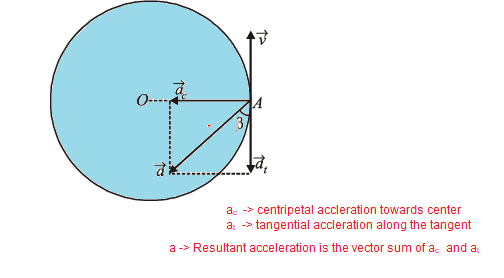As shown above , two accelerations are acting in mutually perpendicular directions.
if $\vec{a}$ be the resultant acceleration, then
$\vec{a} = \vec{a_t} + \vec {a_c}$
Now magnitude is given by
$\left| \vec{a} \right|=\sqrt{a_{t}^{2}+a_{c}^{2}}=\sqrt{\left( 0.5 \right) ^2+\left( 0.7 \right) ^2}=0.86ms^{-2}$
and direction with tangential acceleration vector
$\tan \beta =\frac{a_c}{a_t}=\frac{0.7}{0.5}=1.4$
$\Rightarrow \beta =\tan ^{-1}\left( 1.4 \right) =54.5^0$
So , this is the angle from the direction of negative of the velocity or direction of tangential velocity vector.

Question 4.32
(a) Show that for a projectile the angle between the velocity and the x-axis as a function of time is given by
$\theta \left( t \right) =\tan ^{-1}\left( \frac{v_{oy-}gt}{v_{ox}} \right)$
(b) Show that the projection angle , for a projectile launched from the origin is given by
$\theta _0=\tan ^{-1}\left( \frac{4h_m}{R} \right)$
where the symbols have their usual meaning.
Solution
Let a projectile fired at an angle $\theta$ with x-axis.
As $\theta$ depends on $t$, $\theta (t)$ , at any instant
$\tan \theta \left( t \right) =\frac{v_y}{v_x}=\frac{v_{0y}-gt}{v_{ox}}$
( Since,$v_y=v_{oy}-gt$ and $v_x=v_{ox}$ )
$\theta \left( t \right) =\tan ^{-1}\left( \frac{v_{oy-}gt}{v_{ox}} \right)$
Now $h_{\max}=\frac{u^2\sin ^2\theta}{2g}$
and
$R=\frac{u^2\sin ^2\theta}{g}$
$Rightarrow \frac{h_{\max}}{R}=\frac{\frac{u^2\sin ^2\theta}{2g}}{\frac{u^2\sin ^22\theta}{g}}=\frac{\tan \theta}{4}$

$\left( As\,\,\sin 2\theta =\text{2}\sin \theta \cos \theta \right)$

$\Rightarrow \frac{\tan \theta}{4}=\frac{h_{\max}}{R}$
or,
$\tan \theta =\frac{4h_{\max}}{R}$
or,
$\theta =\tan ^{-1}\left( \frac{4h_{\max}}{R} \right)$